# Water compressibility

Jump to: navigation, search

## Water compressibility

The formation water (brine) isothermal compressibility data is published in 1990 by McCain.

## Math and Physics

At pressures above bubble point: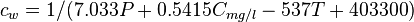$c_w = 1 / (7.033 P + 0.5415 C_{mg/l} - 537 T + 403300)$

## Application range

The correlation is valid only for temperatures between 200 and 270F, pressures of 1000 to 20000 psia, and salinities up to 200000 mg/l.

## Discussion

We, at pengtools.com, are calculating water compressibility below the bubble point with the same equation at the moment.

## Example. Calculating water isothermal compressibility

Example source 

### Input data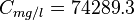$C_{mg/l} = 74289.3$

Calculate water isothermal compressibility at 3500 psia and 165°F?

### Solution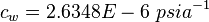$c_w = 2.6348E-6\ psia^{-1}$

The solution is also available in the PVT software model at [The solution is available in the PVT calculator software model at www.pengtools.com

## Nomenclature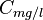$C_{mg/l}$ = pressure correction, res bbl/STB$C_{mg/l}$ = water salinity or solids concentration, mg/l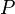$P$ = pressure, psia$T$ = Temperature, °F

## See also

Water bubble point pressure
Water compressibility
Water density
Water formation volume factor
Water salinity from density equation
Water solids concentration
Water viscosity
Gas/Water Interfacial Tension